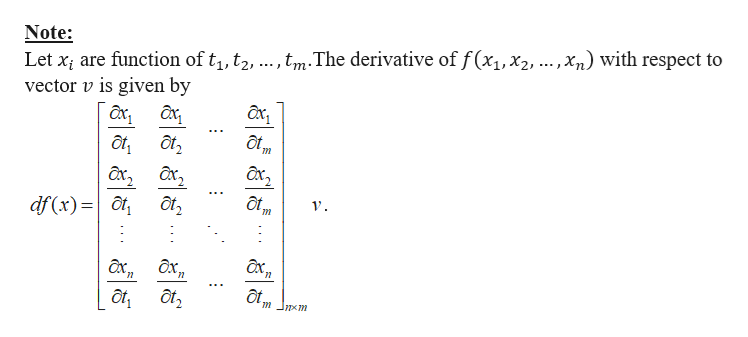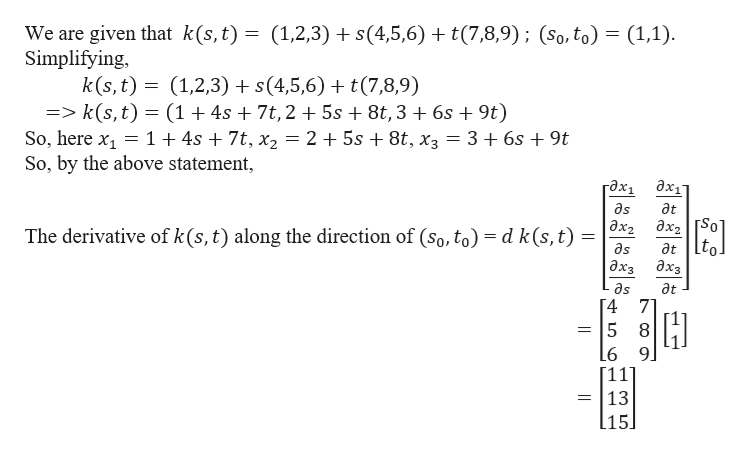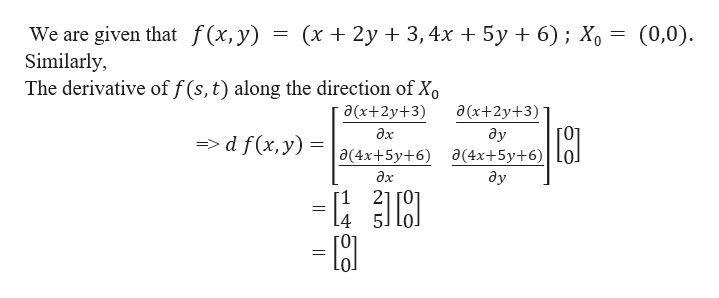# Find the derivative matrix for the functions at the given points. Then findf o k; (s0,t0)=(1,1).a) k(s,t)= (1,2,3)+s(4,5,6)+t(7,8,9) ; (s0,t0)=(1,1)b) f(x,y) = (x+2y+3, 4x+5y+6) ; X0= (0,0)

Question
6 views

Find the derivative matrix for the functions at the given points. Then find

f o k; (s0,t0)=(1,1).

a) k(s,t)= (1,2,3)+s(4,5,6)+t(7,8,9) ; (s0,t0)=(1,1)

b) f(x,y) = (x+2y+3, 4x+5y+6) ; X0= (0,0)

check_circle

Step 1

Let us first write the definition of the derivative matrix for the functions at the given points.help_outlineImage TranscriptioncloseNote: Let xi are function of t1, t2,.,tmThe derivative off(x1,x2,.. ,Xn) with respect to vector v is given by ot ot Ex2 df (x)= ах, дх, ot, శ శ" ఈశే fullscreen
Step 2

For (a),help_outlineImage TranscriptioncloseWe are given that k(s,t) = (1,2,3) s(4,5,6) t(7,8,9); (So, to) = (1,1) Simplifying k(s, t) (1,2,3) s(4,5,6) + t(7,8,9) => k(s,t) (1+4s + 7t,2 + 5s 8t,3 6s + 9t) So, here x1 1+ 4s + 7t, x2 = 2 + 5s + 8t, x3 = 3 + 6s + 9t So, by the above statement гдх, дх1 as дt Әх, дх, So at lto дхз The derivative of k (s, t) along the direction of (so, to) = d k(s, t) as дхз as дt [4 7 5 8 6 9.  = 13 l15] fullscreen
Step 3

For (b),...help_outlineImage Transcriptionclose(х + 2у + 3,4х + 5у + 6); Хо We are given that f(x, y) Similarly The derivative of f(s, t) along the direction of Xo (0,0) a(x+2y+3) Ә(x+2y+3) дх ду => d f(x, y) (4x+5y+6 a(4x+5y+6) дх ду 21 - fullscreen

### Want to see the full answer?

See Solution

#### Want to see this answer and more?

Solutions are written by subject experts who are available 24/7. Questions are typically answered within 1 hour.*

See Solution
*Response times may vary by subject and question.
Tagged in

### Derivative